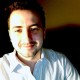ATtiny13 – disco lights using DFT

This is my another “disco lights” project which is an upgraded version of ATtiny13 – dance lights with DFT. This time I used a full implementation of optimized DFT algorithm with reduced memory access to compute a power spectrum of audio signal. This version has also 3-channel lights but visual effects are much-much better!

Circuit DiagramSoftware

This code is written in C and can be compiled using the avr-gcc. All information about how to compile this project is here.

/**
* Copyright (c) 2019, Łukasz Marcin Podkalicki <lpodkalicki@gmail.com>
* ATtiny13/026
* Disco lights using DFT (Discrete Fourier Transformation)
*/

#include <avr/io.h>
#include <avr/interrupt.h>

#define LED_LOW              PB0
#define LED_MID              PB1
#define LED_HIGH             PB2

#define LOW_THRESHOLD        (48000UL)
#define MID_THRESHOLD        (128)
#define HIGH_THRESHOLD       (64)

#define N                    (7) // N-points (DFT)
#define B                    (3 * N / 4) // b-value (DFT)

const int8_t W[N] = {10, 6, -2, -9, -9, -2, 6}; // twiddle factors (DFT)
int8_t samples[N]; // raw samples (ADC)
uint16_t power[N>>1]; // power spectrum (DFT)
volatile uint8_t counter = 0;

static void dft(void);

int
main(void)
{

/* setup */
DDRB |= _BV(LED_LOW)|_BV(LED_MID)|_BV(LED_HIGH); // set LED pins as OUTPUT
sei(); // enable global interrupts

/* loop */
while (1) {
if (counter == N) {
dft(); // do some DSP

/* LOW freqency band */
if (power > LOW_THRESHOLD) {
PORTB |= _BV(LED_LOW);
} else {
PORTB &= ~_BV(LED_LOW);
}

/* MID freqency band */
if (power > MID_THRESHOLD) {
PORTB |= _BV(LED_MID);
} else {
PORTB &= ~_BV(LED_MID);
}

/* HIGH frequency band */
if (power > HIGH_THRESHOLD) {
PORTB |= _BV(LED_HIGH);
} else {
PORTB &= ~_BV(LED_HIGH);
}

counter = 0; // reset samples counter
}
}
}

{

if (counter < N) {
}
}

/**
* Twiddle-factor-based DFT algorithm with reduced memory access.
*/
void
dft(void)
{
uint8_t a, b, i, j;
int16_t re[N];
int16_t im[N];

for (i = 0; i < N; ++i) {
re[i] = 0;
im[i] = 0;
}

for (i = 0; i < (N>>1); ++i) {
a = 0;
b = B;
for (j = 0; j < N; ++j) {
re[i] += W[a%N] * samples[j];
im[i] -= W[b%N] * samples[j];
a += i;
b += i;
}
power[i] = (re[i] * re[i] + im[i] * im[i]) >> 4;
}
}

5 thoughts on “ATtiny13 – disco lights using DFT”

1.jumi

Hi,

please provide hex code and how should fuse settings be

2.Łukasz Podkalicki

Yes, should be fine. I’ve edited this page few days ago and I forgot to change function definition. Sorry for that. Now is up to date.

3.Jack

I use MkCLIPSE
logs:
main.c:45:13: warning: implicit declaration of function ‘dft’ [-Wimplicit-function-declaration]
dft(); // do some DSP
^
main.c: At top level:
main.c:87:1: warning: conflicting types for ‘dft’
dft(void)
^
main.c:45:13: note: previous implicit declaration of ‘dft’ was here
dft(); // do some DSP
^
main.c:26:13: warning: ‘fft’ declared ‘static’ but never defined [-Wunused-function]
static void fft(void);

I fixed the code, but I’m not sure it’s okay (now I can compile)

#include
#include

#define LED_LOW PB0
#define LED_MID PB1
#define LED_HIGH PB2

#define LOW_THRESHOLD (48000UL)
#define MID_THRESHOLD (128)
#define HIGH_THRESHOLD (64)

#define N (7) // N-points (FFT)
#define B (3 * N / 4) // b-value (FFT)

const int8_t W[N] = {10, 6, -2, -9, -9, -2, 6}; // twiddle factors (FFT)
int8_t samples[N]; // raw samples (ADC)
uint16_t power[N>>1]; // power spectrum (FFT)
volatile uint8_t counter = 0;

{

if (counter < N) {
}
}

static void
dft(void)
{
uint8_t a, b, i, j;
int16_t re[N];
int16_t im[N];

for (i = 0; i < N; ++i) {
re[i] = 0;
im[i] = 0;
}

for (i = 0; i >1); ++i) {
a = 0;
b = B;
for (j = 0; j > 4;
}
}

int
main(void)
{

/* setup */
DDRB |= _BV(LED_LOW)|_BV(LED_MID)|_BV(LED_HIGH); // set LED pins as OUTPUT
sei(); // enable global interrupts

/* loop */
while (1) {
if (counter == N) {
dft(); // do some DSP

/* LOW freqency band */
if (power > LOW_THRESHOLD) {
PORTB |= _BV(LED_LOW);
} else {
PORTB &= ~_BV(LED_LOW);
}

/* MID freqency band */
if (power > MID_THRESHOLD) {
PORTB |= _BV(LED_MID);
} else {
PORTB &= ~_BV(LED_MID);
}

/* HIGH frequency band */
if (power > HIGH_THRESHOLD) {
PORTB |= _BV(LED_HIGH);
} else {
PORTB &= ~_BV(LED_HIGH);
}

counter = 0; // reset samples counter
}
}
}

4.Jack

Hi
I have a code problem, my compiler shows a bug in “dft(); // do some DSP ”
How can I solve this?

•Łukasz Podkalicki

Hi Jacek,
could provide some logs describing this error?

Btw. in a december edition an EP (Elektronika Praktyczna) will publish my article about optimized DFT for microcontrollers. If it’s something you would like to dive deeper then I recommend to read it.
/L